# Random Numbers Generation

Generating random numbers on a quantum computer is not difficult because the unpredictability of measure of a qubit in a state of superposition it is in fact a form of natural randomness. Translating this idea into code is relatively simple: taking n qubits (therefore the random number obtained from each execution will be an integer from 0 to 2n - 1) with initial state equals to $|0\rangle$, the quantum Hadamard gate is applied to each of the n qubits and finally the measurement is performed. The quantum Hadamard gate applied to a qubit in $|0\rangle$ state brings it in superposition state: $$\frac{|0\rangle + |1\rangle}{\sqrt{2}}$$ and this means that the measure will have the probability of 50% to be equal to 0 and 50% to be equal to 1.

## Circuit diagram

Considering that a quantum computer with 5 qubits is available, the circuit diagram that achieves the above is the following: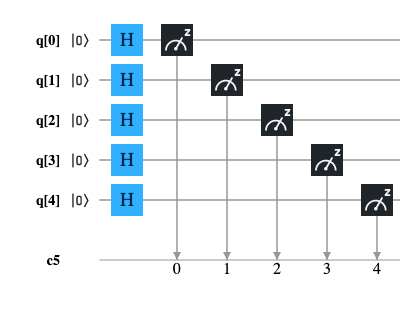## QASM code

The following program is the translation in QASM language of the circuit illustrated above; this program has been tested both on IBM's quantum computer 'ibmq_london' and on the IBM 'qasm_simulator' simulator.

OPENQASM 2.0;
include "qelib1.inc";

qreg q;
creg c;

h q;
h q;
h q;
h q;
h q;

measure q -> c;
measure q -> c;
measure q -> c;
measure q -> c;
measure q -> c;

## Python with Qiskit code

The following program is the translation in Python with Qiskit of the circuit shown above; this program was tested inside a Jupyter Notebook using as backend both IBM's quantum computer 'ibmq_london' and IBM's 'qasm_simulator' simulator.

q = QuantumRegister(5, 'q')
c = ClassicalRegister(5, 'c')
circuit = QuantumCircuit(q, c)

circuit.h(q)
circuit.h(q)
circuit.h(q)
circuit.h(q)
circuit.h(q)

circuit.measure(q, c)

## Microsoft Q# .NET Core code for quantum simulator

The following program is the Microsoft Q# translation of the circuit shown above; this program has been tested on a Linux with .NET Core, Q# runtime and Microsoft quantum simulator installed.

namespace ComputationalMindset.QuantumExperiments

open Microsoft.Quantum.Intrinsic;
open Microsoft.Quantum.Canon;
open Microsoft.Quantum.Measurement;

operation RandomNumberGenerator() : (Result, Result, Result, Result, Result)
{
using (q = Qubit)
{
H(q);
H(q);
H(q);
H(q);
H(q);

let result = (M(q), M(q), M(q), M(q), M(q));

ResetAll(q);
return result;
}
}


## Results on IBM Q Experience

Both programs for IBM Q Experience were executed with a number of shots equals to 8192 both on the IBM's simulator 'qasm_simulator' and on the real computer IBM's 'ibmq_london' obtaining the expected result, ie a uniform distribution of all 5-bit combinations. The imperfect uniformity shown in the case of real quantum computers compared to simulators is due to noise.
Note: Given the stochastic nature of this code, your specific reults may vary. Consider running the example a few times.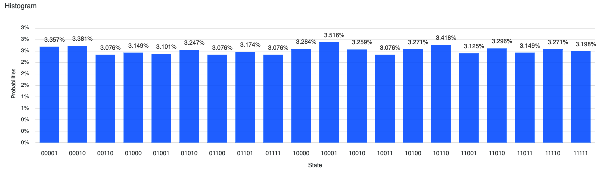QASM program execution result on IBM simulator 'qasm_simulator' (first 20 combinations)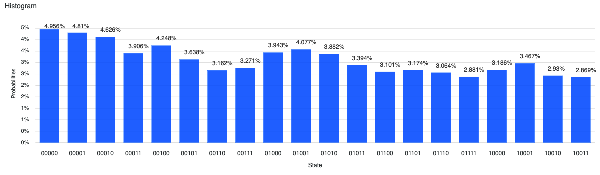QASM program execution result on IBM real quantum computer 'ibmq_london' (first 20 combinations)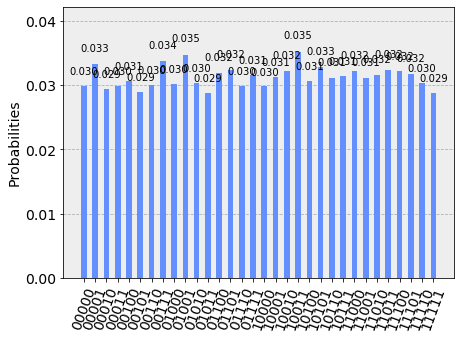Python + Qiskit program execution result on IBM simulator 'qasm_simulator'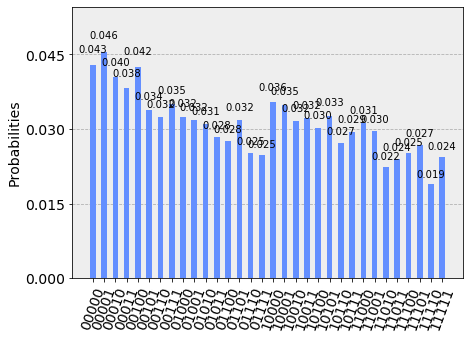Python + Qiskit program execution result on IBM real quantum computer 'ibmq_london'

## Results on Microsoft simulator

The execution of the Q# program for .NET Core produced the expected result, namely a uniform distribution of all 5 bits combinations.
Note: Given the stochastic nature of this code, your specific results may vary. Consider running the example a few times.

Binary Decimal Count Probability (%)
00000002573.14%
00001012653.24%
00010022402.93%
00011032553.11%
00100042372.89%
00101052653.24%
00110062673.26%
00111072553.11%
01000082573.14%
01001092563.13%
01010102412.94%
01011112463.00%
01100122513.06%
01101132643.22%
01110142723.32%
01111152653.24%
10000162232.72%
10001172442.98%
10010182623.20%
10011192452.99%
10100202553.11%
1010121/td>2483.03%
10110222513.06%
10111232563.13%
11000242723.32%
11001252673.26%
11010262923.56%
11011272272.77%
11100282783.39%
11101292623.20%
11110302573.14%
11111312603.17%
Totals: 8192 100.00%
Q# program result on Linux with .NET Core and Microsoft quantum simulator